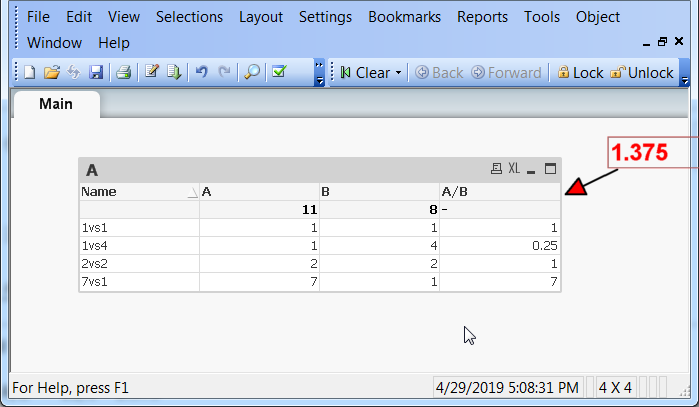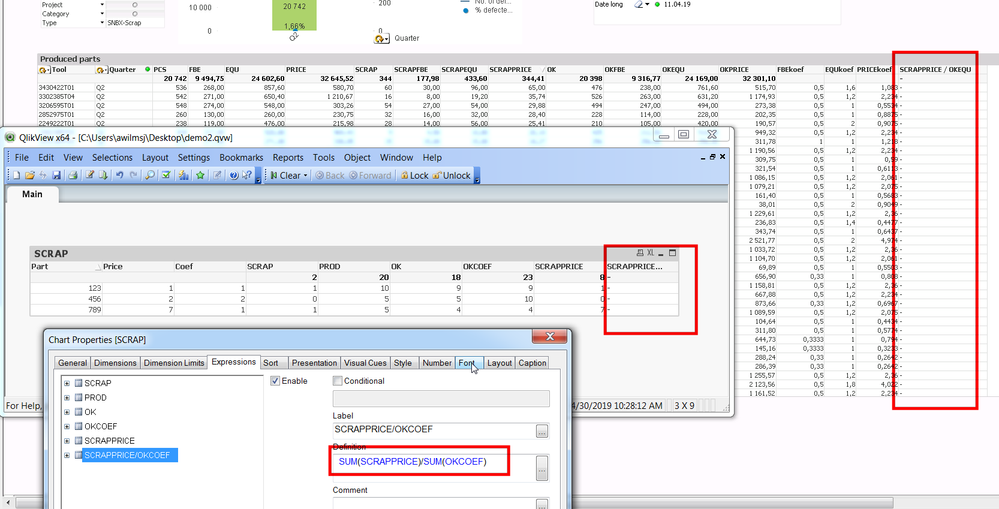# QlikView App Dev

Discussion Board for collaboration related to QlikView App Development.

Announcements
Join this live chat April 6, 10AM EST - QlikView to Qlik Sense REGISTER
cancel
Showing results for
Search instead for
Did you mean:Contributor

## Chart Row expression vs Totals Mode issue

Dears,

I hope the screenshot is self explaining.

My expression for column C is simply deviding 2 columns A and B by eachother

The Total lines of column A and B are set to sum.

How to get the Total line of C to match the total of A / total of B.

I have tried with sum aggr total... but fail to get the result i am looking for.

thanks,

Jan1 Solution

Accepted SolutionsCreator III

Hi

Can you please use the expression below

=If(Dimensionality() = 0,Sum(Total Aggr(SUM({<Type={'SCRAP'}>}Qty)*Price,Part,Coef)) / Sum(Total Aggr((SUM({<Type={'Produced'}>}Qty) - SUM({<Type={'SCRAP'}>}Qty))*Coef,Part,Coef)),SCRAPPRICE/OKCOEF)

Thanks

Kushal

13 RepliesPartner

Your screen shot is corrupted. Upload a new one.

From my understanding, you can use Expression total or sum of rows in Expression tab Total Mode option.

You Know What To Do.Contributor
Author

Does this work ?Partner

Instead of sum of Rows. Use the Above option Expression Total

You Know What To Do.Contributor
Author

I tried "Expression Total" already.

Its resulting in a '-' as total.Contributor
Author

Adding a simplified QVW

How to get the totals of A/B --> 11/8 = 1.375Partner

Is this what you want?

Also you can use Column(1)/Column(2)

You Know What To Do.Contributor
Author

Unfortunately real life is a bit more complex than the example i attached previously.

I tried to make my demo qvw 1 step closer to what i have in reality...

Putting the SUM(A)/SUM(B) as you proposed is returning "-" in the sample attached same as it does in my real life scenario.

See attached.Contributor
Author
(SUM({<Type={'SCRAP'}>}Qty)*Price)/((SUM({<Type={'Produced'}>}Qty)-SUM({<Type={'SCRAP'}>}Qty))*Coef)

This is the last expression fully written down.
If i add Sum function around nominator and denominator
SUM(SUM({<Type={'SCRAP'}>}Qty)*Price)/SUM((SUM({<Type={'Produced'}>}Qty)-SUM({<Type={'SCRAP'}>}Qty))*Coef)
the entire table goes blank... you can try on the demo2.qvw attached in post above.
Challenge remains to get the totals of the last column to match this expression.Creator III

Hi Jan

you can use below expression for the attached qvw

If(Dimensionality() = 0,sum( total A)/sum(total B),Sum(A)/Sum(B))

Thanks

Kushal